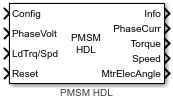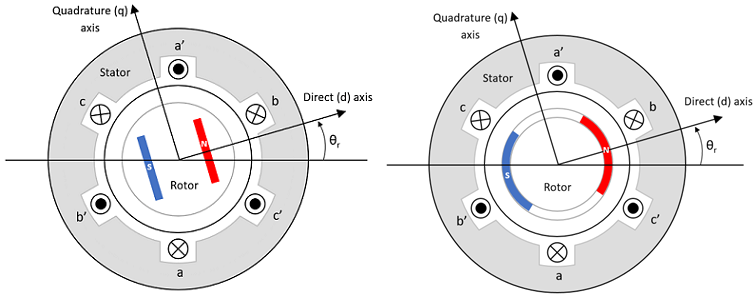# PMSM HDL

Three-phase permanent magnet synchronous motor with sinusoidal back electromotive force

Since R2022b

•Libraries:
Motor Control Blockset HDL Support / Electrical Systems / Motors

## Description

The PMSM HDL block implements a three-phase permanent magnet synchronous motor (PMSM) with a sinusoidal back-electromotive force. The block uses three-phase input voltages to regulate the individual phase currents, thereby controlling the motor torque or speed.

The block generates code for fixed-step double- and single-precision targets using the Sample Time (s) parameter. It supports code generation for FPGA deployment and generates HDL-compatible code.

You can specify the PMSM parameters and operating mode using the Config input port. You can also use the PMSM Configuration block to generate the required configuration signal to specify at the Config input port.

### Motor Construction

These diagrams show the interior and surface-mount PMSM construction with a single-pole pair on the motor.The motor magnetic field due to the permanent magnets creates a sinusoidal rate of change of flux with the motor angle.

For the axes convention, the phase-a aligns with d-axis when the motor angle θr is zero.

### Three-Phase Sinusoidal Model Electrical System

The block implements these equations expressed in the motor flux reference frame (dq frame). All quantities in the motor reference frame are referred to as the stator.

`$\begin{array}{l}{\omega }_{e}=P{\omega }_{m}\\ \frac{d}{dt}{i}_{d}=\frac{1}{{L}_{d}}{v}_{d}-\frac{R}{{L}_{d}}{i}_{d}+\frac{{L}_{q}}{{L}_{d}}P{\omega }_{m}{i}_{q}\end{array}$`

`$\frac{d}{dt}{i}_{q}=\frac{1}{{L}_{q}}{v}_{q}-\frac{R}{{L}_{q}}{i}_{q}-\frac{{L}_{d}}{{L}_{q}}P{\omega }_{m}{i}_{d}-\frac{{\lambda }_{pm}P{\omega }_{m}}{{L}_{q}}$`

`${T}_{e}=1.5P\left[{\lambda }_{pm}{i}_{q}+\left({L}_{d}-{L}_{q}\right){i}_{d}{i}_{q}\right]$`

The Lq and Ld inductances represent the relation between the phase inductance and the motor position due to the saliency of the motor magnets. For a surface mount PMSM, ${L}_{d}={L}_{q}$.

The equations use these variables.

 Lq, Ld q- and d-axis inductances (H) R Resistance of the stator windings (ohm) iq, id q- and d-axis currents (A) vq, vd q- and d-axis voltages (V) ωm Angular mechanical velocity of the motor (rad/s) ωe Angular electrical velocity of the motor (rad/s) λpm Permanent magnet flux linkage (Wb) Ke Back electromotive force (EMF) (Vpk_LL/krpm, where Vpk_LL is the peak voltage line-to-line measurement) Kt Torque constant (N·m/A) P Number of pole pairs Te Electromagnetic torque (Nm) Θe Electrical angle (rad)

### Mechanical System

The motor angular velocity is given by:

`$\begin{array}{c}\frac{d}{dt}{\omega }_{m}=\frac{1}{J}\left({T}_{e}-{T}_{f}-F{\omega }_{m}-{T}_{m}\right)\\ \frac{d{\theta }_{m}}{dt}={\omega }_{m}\end{array}$`

The equations use these variables.

 J Combined inertia of motor and load (kgm^2) F Combined viscous friction of motor and load (N·m/(rad/s)) θm Motor mechanical angular position (rad) Tm Motor shaft torque (Nm) Te Electromagnetic torque (Nm) Tf Motor shaft static friction torque (Nm) ωm Angular mechanical velocity of the motor (rad/s)

### Power Accounting

For motor power accounting, the block implements these equations.

Bus Signal DescriptionVariableEquations

`PwrInfo`

`PwrTrnsfrd` — Power transferred between blocks

• Positive signals indicate flow into block

• Negative signals indicate flow out of block

`PwrMtr`

Mechanical power

Pmot

`PwrBus`

Electrical power

Pbus

`PwrNotTrnsfrd` — Power crossing the block boundary, but not transferred

• Positive signals indicate an input

• Negative signals indicate a loss

`PwrElecLoss`

Resistive power loss

Pelec

`PwrMechLoss`

Mechanical power loss

Pmech

When Port Configuration is set to `Torque`:

When Port Configuration is set to `Speed`:

`PwrStored` — Stored energy rate of change

• Positive signals indicate an increase

• Negative signals indicate a decrease

`PwrMtrStored`

Stored motor power

Pstr

The equations use these variables.

 Rs Stator resistance (ohm) ia, ib, ic Stator phase a, b, and c current (A) isq, isd Stator q- and d-axis currents (A) van, vbn, vcn Stator phase a, b, and c voltage (V) ωm Angular mechanical velocity of the motor (rad/s) F Combined motor and load viscous damping N·m/(rad/s) Te Electromagnetic torque (Nm) Tf Combined motor and load friction torque (Nm)

## Ports

### Input

expand all

Configuration signal for the PMSM HDL block containing the block configuration parameters. For more information about the constituent parameters, see the Config output of the PMSM Configuration block.

Data Types: `single`

Stator terminal voltages Va, Vb, and Vc, in V.

Data Types: `single`

Based on the mode of operation defined by the Port Configuration parameter available in the multiplexed Config input, the port supports one of these inputs:

• Tm — Load torque on the motor shaft, in N·m.

• ωm — Angular velocity of the motor, in rad/s.

Data Types: `single`

Reset bus signal that the block uses to reset the internal integrators.

Data Types: `Boolean`

### Output

expand all

The bus signal contains these block calculations.

Signal DescriptionVariableUnits

`IaStator`

Stator phase current A

ia

A

`IbStator`

Stator phase current B

ib

A

`IcStator`

Stator phase current C

ic

A

`IdSync`

Direct axis current

id

A

`IqSync`

iq

A

`VdSync`

Direct axis voltage

vd

V

`VqSync`

vq

V

`MtrSpd`

Angular mechanical velocity of the motor

ωm

`MtrPos`

Motor mechanical angular position

θm

`MtrTrq`

Electromagnetic torque

Te

N·m

`PwrInfo`

`PwrTrnsfrd`

`PwrMtr`

Mechanical power

Pmot

W
`PwrBus`

Electrical power

Pbus

W

`PwrNotTrnsfrd`

`PwrElecLoss`

Resistive power loss

Pelec

W
`PwrMechLoss`

Mechanical power loss

Pmech

W

`PwrStored`

`PwrMtrStored`

Stored motor power

Pstr

W

Current for phase a, b, and c, ia, ib, and ic, respectively, in A.

Motor torque, Tmtr, in N·m.

Angular speed of the motor, ωmtr, in rad/s.

Electrical position of the motor, θe, in rad.

Data Types: `single`

## Parameters

expand all

The fixed time interval (in seconds) between consecutive instances of block execution. Alternatively, you can use `-1`, to inherit the sample time from the input signals.

## Version History

Introduced in R2022b